# Solution assignment 08 Logarithmic equations

### Assignment 8

Solve: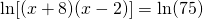### Solution

The domain of the function in the left-hand side is: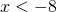or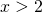We rewrite the equation: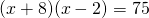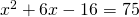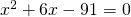The solution of this equation is: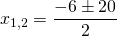thus: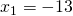or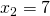Both solutions lie in the domain and are valid solutions.

Note. Compare this equation with the following assignment.

Solve: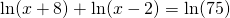In this case the domain of the function in the left-hand side is: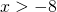andthus:We proceed in the same way as before, with as result the solutions:orHowever, now the first solution does not satisfy the equation because it lies outside the domain.